Area of sector of circle

Chapter 12 Class 10 Areas related to Circles
Concept wise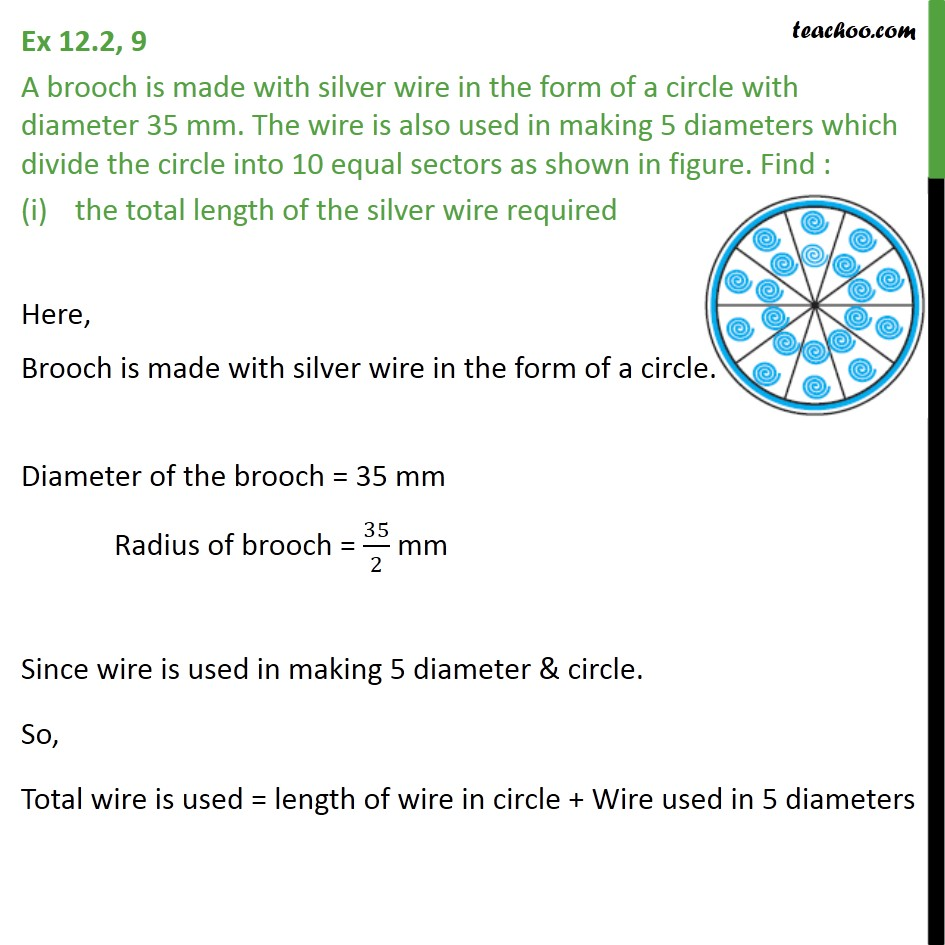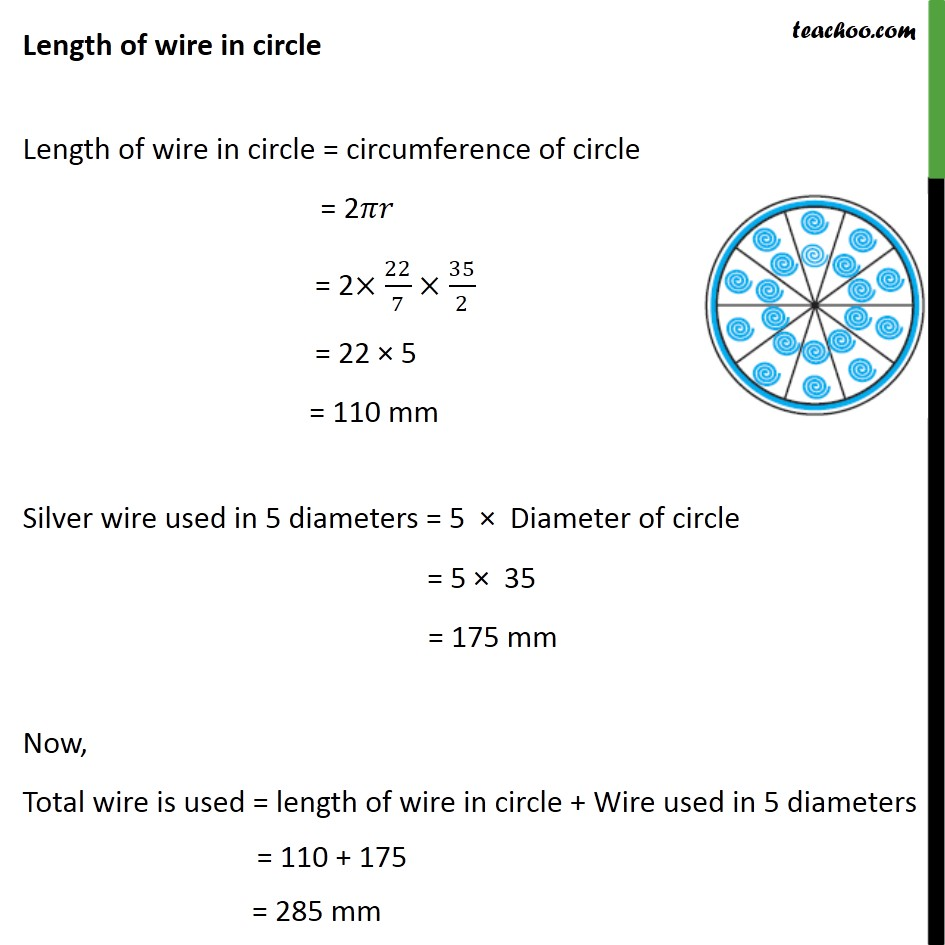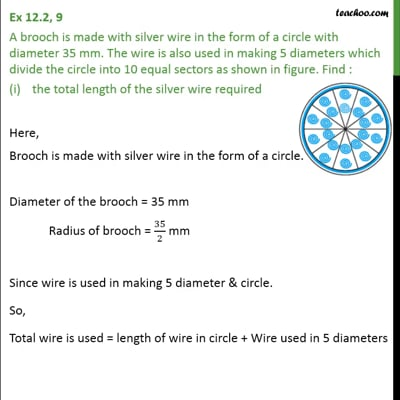This video is only available for Teachoo black users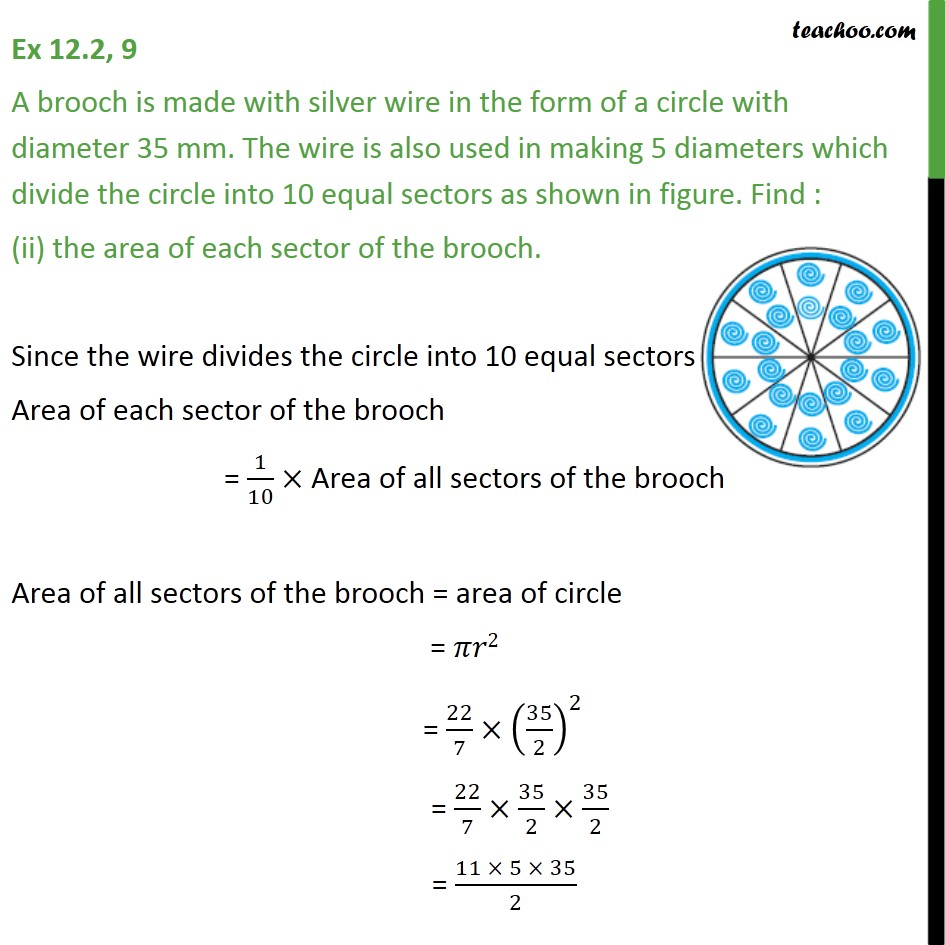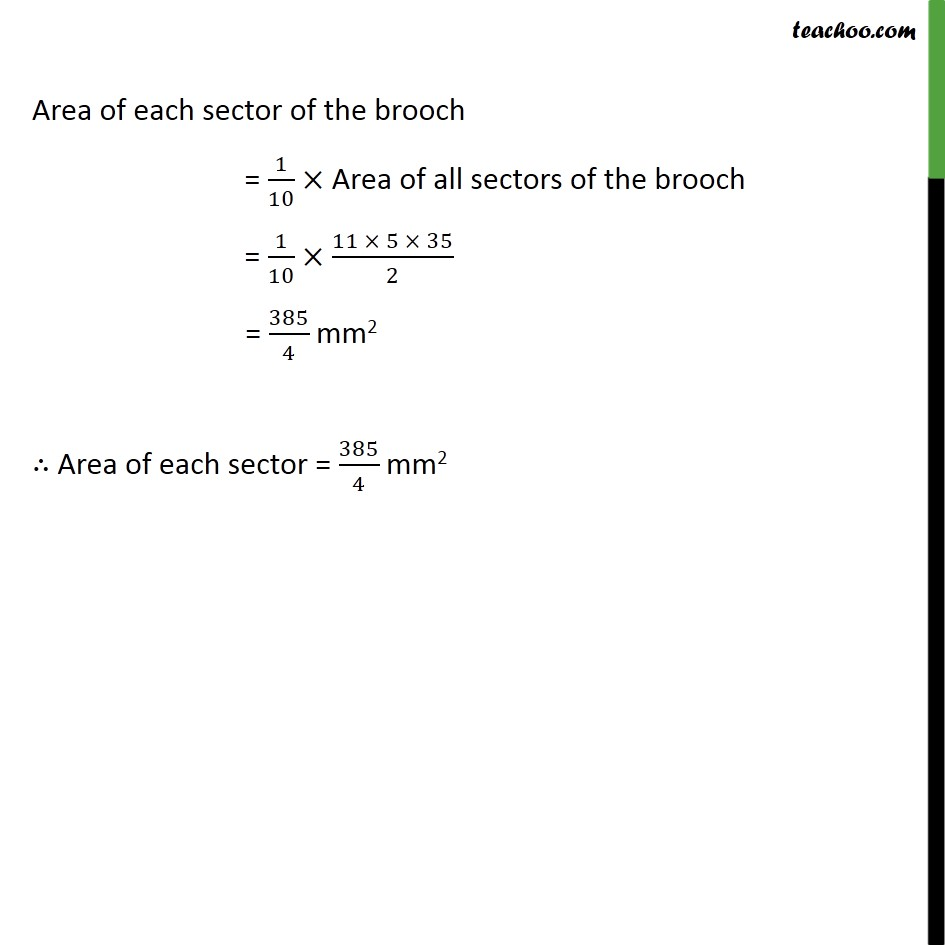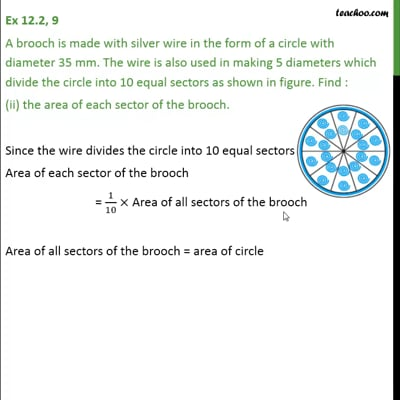This video is only available for Teachoo black users

Solve all your doubts with Teachoo Black (new monthly pack available now!)

### Transcript

Ex 12.2, 9 A brooch is made with silver wire in the form of a circle with diameter 35 mm. The wire is also used in making 5 diameters which divide the circle into 10 equal sectors as shown in figure. Find : the total length of the silver wire required Here, Brooch is made with silver wire in the form of a circle. Diameter of the brooch = 35 mm Radius of brooch = 35/2 mm Since wire is used in making 5 diameter & circle. So, Total wire is used = length of wire in circle + Wire used in 5 diameters Length of wire in circle Length of wire in circle = circumference of circle = 2 = 2 22/7 35/2 = 22 5 = 110 mm Silver wire used in 5 diameters = 5 Diameter of circle = 5 35 = 175 mm Now, Total wire is used = length of wire in circle + Wire used in 5 diameters = 110 + 175 = 285 mm Ex 12.2, 9 A brooch is made with silver wire in the form of a circle with diameter 35 mm. The wire is also used in making 5 diameters which divide the circle into 10 equal sectors as shown in figure. Find : (ii) the area of each sector of the brooch. Since the wire divides the circle into 10 equal sectors Area of each sector of the brooch = 1/10 Area of all sectors of the brooch Area of all sectors of the brooch = area of circle = 2 = 22/7 (35/2)^2 = 22/7 35/2 35/2 = (11 5 35)/2 Area of each sector of the brooch = 1/10 Area of all sectors of the brooch = 1/10 (11 5 35)/2 = 385/4 mm2 Area of each sector = 385/4 mm2